##### Pre-Calculus All-in-One For DummiesWant some good news, free of charge? Graphing parent functions and transformed logs is a snap! You can change any log into an exponential expression, so this step comes first. You then graph the exponential, remembering the rules for transforming, and then use the fact that exponentials and logs are inverses to get the graph of the log.

## How to graph a parent function

Exponential functions each have a parent function that depends on the base; logarithmic functions also have parent functions for each different base. The parent function for any log is written f(x) = logb x. For example, g(x) = log4 x corresponds to a different family of functions than h(x) = log8 x. This example graphs the common log: f(x) = log x.
1. Change the log to an exponential.

Because f(x) and y represent the same thing mathematically, and because dealing with y is easier in this case, you can rewrite the equation as y = log x. The exponential equation of this log is 10y = x.

2. Find the inverse function by switching x and y.

You find the inverse function 10x = y.

3. Graph the inverse function.

Because you’re now graphing an exponential function, you can plug and chug a few x values to find y values and get points. The graph of 10x = y gets really big, really fast. You can see its graph in the figure.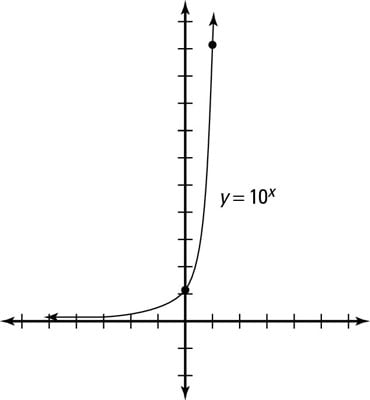y = 10x."/>
Graphing the inverse function y = 10x.
4. Reflect every point on the inverse function graph over the line y = x.

The next figure illustrates this last step, which yields the parent log’s graph.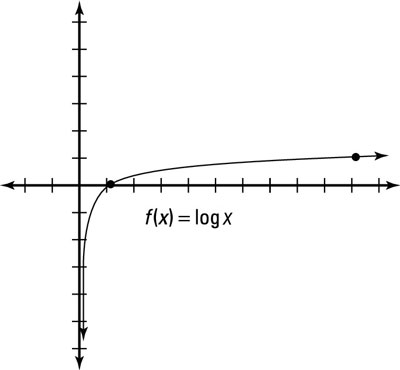f(x) = log x."/>
Graphing the logarithm f(x) = log x.

## How to graph a transformed log

All transformed logs can be written as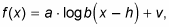where a is the vertical stretch or shrink, h is the horizontal shift, and v is the vertical shift.

So if you can find the graph of the parent function logb x, you can transform it. However, most students still prefer to change the log function to an exponential one and then graph. The following steps show you how to do just that when graphing f(x) = log3(x – 1) + 2:

1. Get the logarithm by itself.

First, rewrite the equation as y = log3(x – 1) + 2. Then subtract 2 from both sides to get y – 2 = log3(x – 1).

2. Change the log to an exponential expression and find the inverse function.

If y – 2 = log3(x – 1) is the logarithmic function, 3y – 2 = x – 1 is the exponential; the inverse function is 3x – 2 = y – 1 because x and y switch places in the inverse.

3. Solve for the variable not in the exponential of the inverse.

To solve for y in this case, add 1 to both sides to get 3x – 2 + 1 = y.

4. Graph the exponential function.

The parent graph of y = 3x transforms right two (x – 2) and up one (+ 1), as shown in the next figure. Its horizontal asymptote is at y = 1.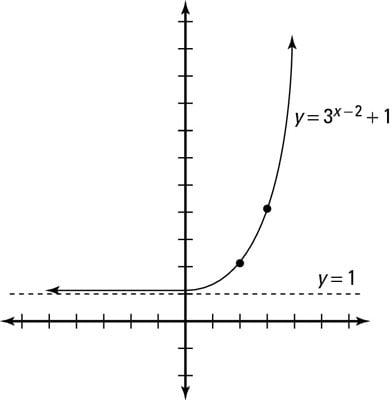The transformed exponential function.
5. Swap the domain and range values to get the inverse function.

Switch every x and y value in each point to get the graph of the inverse function. The next figure shows the graph of the logarithm.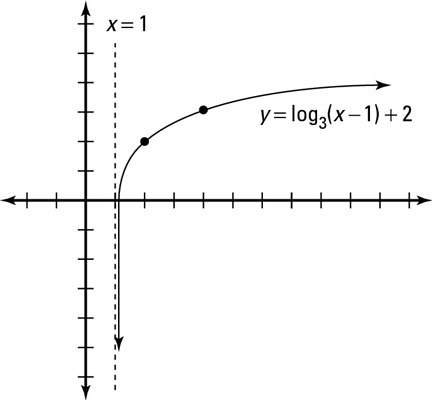You change the domain and range to get the inverse function (log).
Did you notice that the asymptote for the log changed as well? You now have a vertical asymptote at x = 1. The parent function for any log has a vertical asymptote at x = 0. The function f(x) = log3(x – 1) + 2 is shifted to the right one and up two from its parent function p(x) = log3 x (using transformation rules), so the vertical asymptote is now x = 1.